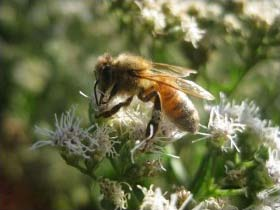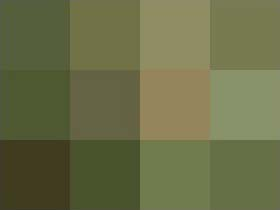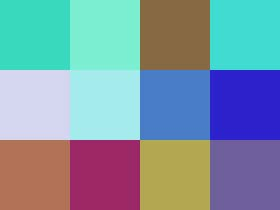Technical Note of "Split/Merge AudioVisual" 1 Artist: Kenji Kojima Technical Note 1, 2, 3   This note is under CC License BY-NC-SA 3.0 index@kenjikojima.comPlease consider making a donation to support Kenji Kojima's works
This technical note uses LiveCode programming.
You can download free community edition LiveCode 6.1.3 (recommend under this version). Over 6.6.0 cannot make a mosaic.

Binary data of an image
When we treat computer files of audio and visual in binary, they do not have borders. You can handle them like same sense of files. Binary is like a pigment which is one of basic materials for an artist. The original image (left) converted to a mosaic (right). The mosaic cells are made large and small numbers for the explanation of this note. You should think the mosaic cells are pixels. The horizontal pixels are 4 and the vertical pixels are 3. Each pixel contains 4 binary data. The binaries of the top left green pixel are "00000000, 01010110, 01011111, 00111011". The first binary is alpha or mask data, the second is "Red", the third is "Green" and the fourth is "Blue" data. The last three binaries are called RGB color values in general. There are 12 pixels in the mosaic image. One each pixel has 4 binary data. The total is 4 by 12 that is 48 binary data in the mosaic image. You can get ASCII codes of the image data by using the word "imagedata" in LiveCode programming.```
get the imagedata of image "mosaic"
-- actual size of image "mosaic" is 4 pixels by 3 pixels
-- the binary data of 12 pixels are "V_;prFéçbw{ROW2dcEïÑ\àëj@<!JR-q{MfpF" which is ASCII.

-- convert ASCII of the first cell (pixel) to decimal (base 10)
put character 1 to 4 of it into firstCell  -- this is only the first cell
repeat with i=1 to 4
put charToNum(character i of firstCell) & space after tDecimal
end repeat
-- the result of tDecimal contains "0 86 95 59 "

```
The first number 0 of the result is the alpha or the mask data. The second number 86 is Red color value, the third 95 is Green color value and the forth 59 is Blue color value of the first image cell (pixel). The numbers are called RGB color. BRG color values are assigned from 0 to 255 by 10 base numbers. Decimal 255 is described 8 digit number "11111111". Sometimes RGB values are used hexadecimal (base 16), however we will use 8 digit binary for cryptography in here.

A bitwise XOR can split an image to two image data. It is one of cipher technologies. If you prefer a correct expression, it is not a splitting image. But we can get same length of two data consequently, and convert them to two musics. It looks separating two data then I prefer a word "split" in this project. We make a random number for each pixel element. You have to make 4 random digital numbers for 1 pixel. The numbers are used a key of cipher. The bitwise operation performs directly on the bits of two numbers. Bitwise XOR makes 0 and 0 to 0, 0 and 1 to 1, 1 and 1 to 0. But the position of the decimal does not change. If 8 bits binary 1 is 10010011 and 8 bits binary 2 is 00100001, the result will be 10110010.
```0 and 0 -> 0
0 and 1 -> 1
1 and 0 -> 1
1 and 1 -> 0

10010011 (binary 1)
00100001 (binary 2)
------------------------
10110010 (the result)
```

Now we have 4 numbers "0 86 95 59" of the first pixel. The first decimal number "0" is "00000000" as 8 digit binary. And I made random decimal "20" that is "00010100" as as 8 digit binary.
```
00000000 (the first number in the pixel. 0 in decimal)
00010100 (random number 20 in decimal)
----------------------------------------
00010100 (the result is 20 in decimal)
```

The second decimal number "86" is "01010110" as 8 digit binary. And I made random decimal "14" that is "00001110" as as 8 digit binary.
```
01010110 (the second number in the pixel. 86 in decimal)
00001110 (random number 14 in decimal)
----------------------------------------
01011000 (the result is 88 in decimal)
```

Fortunately we can use decimals for a bitwise operation in LiveCode programming. These are 2 examples we evaluated above.
```-- LiveCode expression
0 bitXOr 20
-- evaluates to 20
86 bitXOr 14
-- in binary: 1010110 bitXOr 0001110; evaluates to 1011000; converts to 88
```

When a bitwise XOR operation takes in the opposite way, like "20 bitXOr 20" evaluates "0". And "88 bitXOr 14" evaluates "86". You can get original numbers. We will use these processes for merging musics to an original image.
```
00010100 (the result is 20 in decimal)
00010100 (random number 20 in decimal)
--------------------------------------------
00000000 (the first number in the pixel)

01011000 (the result is 88 in decimal)
00001110 (random number 14 in decimal)
---------------------------------------------
01010110 (the second number in the pixel that is 86 in decimal)
```

I just explained a basic method of an encryption and a decryption technology of this project. We operate this method on every each binary data by repeat control structure. This is one of cipher technologies and called the one-time pad. It has been mathematically proven to be uncrackable if it is used correctly. The result of ciphertext and a key of a one-time pad should be a secret and the key is used only once in an actual cipher. However the project is not a concealed purpose. The result of cipherimage and a key are converted musics and disclosed in audiences. Because my purpose is erasing boundaries of two difference senses.

RGB Values and a Musical Note
We make two musics from the two data. One of data is random numbers that same length with a target image. Random numbers is a key of encryption. It encrypts an image and decrypts to an original image. RGB value is from 0 to 255. In the early Macintosh days we used HyperCard. It played a music by numbers and the decimal 60 was the middle C. I supposed RGB value 120 should be the middle C. It is two times of 60. A midi can play twelve-tone scale music. Every 2 value changes a half step of the 12 tone scale.
for converting a number to a musical note. You can download a LiveCode library "makeSMF" from UDI's site.

Random Numbers Key
We have to make random numbers which are same length of pixels of an image (mosaic). We already knew the image data of this. The ramdom numbers must be same length of the imagedata of the image. However low numbers of RGB value are converted to too low sounds. I use the number from 11 to 254. The function "randomNumbers" returns a random number from 11 to 254.
```-- the function to get a random number
function randomNumbers
put random(244) + 10 into tNumber
return tNumber
end rrandomNumbers

-- the function to get mosaic data from an original image
-- the original image name is "pOriginal", the mosaic width is "pMosaicWidth"
function getMosaicData pOriginal, pMosaicWidth
put the rect of image pOriginal into tRect
put the formattedWidth of image pOriginal into tOriginalWidth
put the formattedHeight of image pOriginal into tOriginalHeight
set the width of image pOriginal to pMosaicWidth
set the height of image pOriginal to pMosaicWidth * tOriginalHeight div tOriginalWidth
put the imagedata of image pOriginal into tMosaicData
set the rect of image pOriginal to tRect
return tMosaicData
end getMosaicData

put the length of getMosaicData(tOriginal, 4) into mosaicLength
-- the mosaic image data is "V_;prFéçbw{ROW2dcEïÑ\àëj@<!JR-q{MfpF" which is ASCII.

-- random numbers have to be made the length of the mosaic data (the number of pixels by 4)
repeat with i=1 to mosaicLength
put randomNumbers() & space after tRandomNums
end repeat
put tRandomNums   -- the random numbers (every time it makes different numbers)
```

Cipher Image
You can get the image data of a mosaic by the function "getMosaicData". But you have to convert them to ASCII numbers. Then bitwise XOR performs mosaic image data numbers by random numbers by the length of the mosaic image data times of repeat control structure.

```put getMosaicData(tOriginal, 4) into tMosaicData
-- tMosaicData contains the imagedata of the mosaic image
-- tOiginal is the name of original image, 4 is the width of mosaic image
put the length of tMosaicData into tMosaicLength
-- the length of the mosaic image data

repeat with i=1 to tMosaicLength step 4   -- convert by each pixel
if char i of tMosaicData is empty then exit repeat
put charToNum(char i of tMosaicData) bitXOr (word i of tRandomNums)  into tNum1
put charToNum(char i + 1 of tMosaicData) bitXOr (word i + 1 of tRandomNums) into tNum2
put charToNum(char i + 2 of tMosaicData) bitXOr (word i + 2 of tRandomNums) into tNum3
put charToNum(char i + 3 of tMosaicData) bitXOr (word i + 3 of tRandomNums) into tNum4
put tNum1 & space & tNum2 & space & tNum3 & space & tNum4 & space after tMosaicNums
end repeat
put tMosaicNums   -- the ciphered mosaic numbers
```

The key image and ciphered image
You do not need this step, but you can make images of a key and a ciphered images from tRandomNums and tMosaicNums. Make 2 image objects, the image name is "pix1" and the image name is "pix2". Both of image objects are same width and height of image "tOriginal".

```command setKeyCipherImage pImagedataNum, pImagePixNum, pMosaicWidth
put the num of words of pImagedataNum into tNum
repeat with i=1 to tNum
put numToChar(word i of pImagedataNum) after tData
end repeat
put the rect of image ("pix" & pImagePixNum) into tRect
put the width of image ("pix" & pImagePixNum) into tOriginalWidth
put the height of image ("pix" & pImagePixNum) into tOriginalHeight
set the width of image ("pix" & pImagePixNum) to pMosaicWidth
set the height of image ("pix" & pImagePixNum) to pMosaicWidth * tOriginalHeight div tOriginalWidth
set the imagedata of image ("pix" & pImagePixNum) to tData
set the rect of image ("pix" & pImagePixNum) to tRect
end setKeyCipherImage

setKeyCipherImage tRandomNums, 1, 4  -- the image of random number key
setKeyCipherImage tMosaicNums, 2, 4  -- the image of ciphered mosaic
```image "pix1" numbers (random numbers key): 140 11 217 190 84 123 238 209 186 136 105 66 246 63 219 208 156 212 213 240 118 165 235 236 249 73 125 198 230 46 34 204 229 176 114 85 90 155 39 102 16 179 168 81 31 110 95 155image "pix2" numbers (ciphered mosaic image): 140 93 134 133 84 11 156 151 186 6 228 32 246 72 160 130 156 155 130 194 118 193 136 169 249 220 249 154 230 166 179 166 229 240 78 116 90 209 117 75 16 194 211 28 31 8 47 221

Examination
Before I go to converting colors to musical notes, I would like to exam the decryption using the random numbers key and the ciphered mosaic image. We have 2 variables "tRandomNums" and "tMosaicNums". And we have to make an image object "exam" which is same size as image "mosaic". The decrypted data will be set to image "exam".

```put 1 into tCount
repeat for each word tWord in tRandomNum
put numToChar(tWord bitXor (word tCount of tMosaicNum)) after tImageDatathe binary data of image "exam" V_;prFéçbw{ROW2dcEïÑ\àëj@